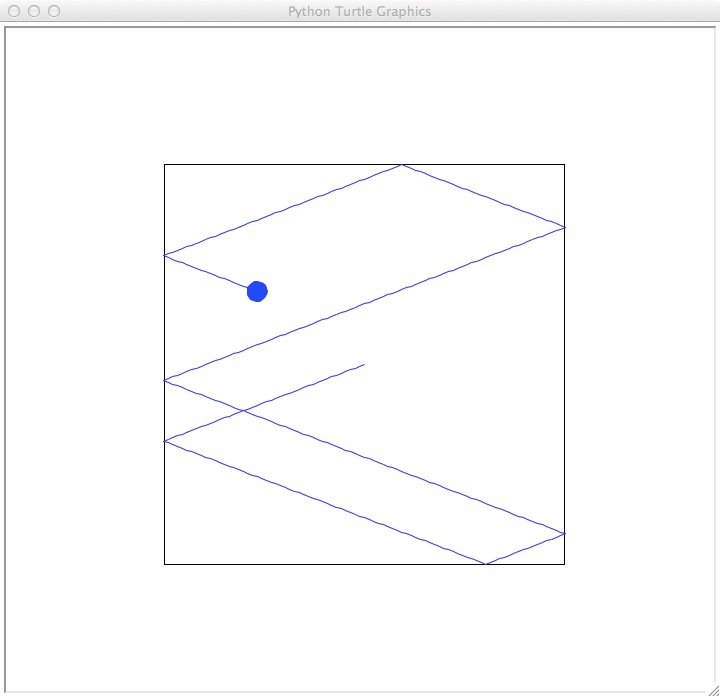This lab builds a simple class for billiard balls, using the built-in turtle library.

As the class has progressed, we have been using classes and objects that others have written. For example, Zelle (the author of our textbook) defined a series of classes for graphics (that reside in graphics.py file). In today's lab, we will define our own class and instantiate objects for it.

We're going to write a simple class to represent billiard (pool) balls that bounce off the boundary edges (i.e. the edges of the billiard table). For this simple class, we will focus on just single objects and not the interaction between them (or multitasking-- it's beyond the scope of this course but will be covered next semester).

In the image below is an example of a billiard ball in action. the billiard ball is blue with boundaries of the bounding box of (-200,-200) to (200,200). The ball started at the center pointed in a random direction and travelled for 400 iterations, bouncing off boundary walls when it encountered them:Here is the main() program:

def main():
setUpTable(-200,-200,400)

b1 = TurtleBall("blue",random()*360,-200,-200,200,200)
b1.move(400)

turtle.Screen().exitonclick()
main()

The function setUpTable draws the boundaries of the billiard table to the screen given the minimal x and y coordinates and the length of the sides. b1 is an object of our new class turtleBall.

Before we go into the details of the class:

2. Experiment with the program by adding a new object, b2 to the main program that has a billiard ball that is green:
b2 = TurtleBall("green",random()*360,-100,-100,100,100)
b2.move(400)

And run your program. What happens? Why does it only cover part of the screen? What is different from the definition of b1 and b2?
3. Add a third billiard ball that bounces outside of table drawn to the screen.

Our class will have instance variables (to keep track of properties of each object) and methods (functions that can be applied to the objects). For instance variables, we will use a turtle to keep track of the location and color. For methods, we need to write a constructor method (called __init__ in Python) that creates new objects of the class. Note that in our main program, we invoke a class method called move() that moves the billiard ball around the screen. Now, let's look at the class definition in detail. In Python, classes begin with the keyword, class:

#A simple class, demonstrating a constructor and methods in python
#Intro Programming, Lehman College, CUNY, Spring 2014
from turtle import *

class TurtleBall:
Classes contain constructor methods that are used to create new objects. Constructors are always called __init__ and all methods in a class have as their first parameter the object itself (traditionally called self). Our class has a constructor that has input parameters: the object itself, the starting color and angle, and the boundaries for where it can go. It then creates a turtle (called self.turtle) as an instance variable, as well as 4 instance variables for storing the bounding box of the boundary (self.xMin, self.yMin, self.xMax, and self.yMax):
def __init__(self,color,angle,x1,y1,x2,y2):
self.turtle = Turtle()
self.turtle.color(color)
self.turtle.shape("circle")
self.turtle.left(angle)
self.turtle.speed(0)
self.xMin,self.yMin,self.xMax,self.yMax = x1,y1,x2,y2

The most interesting part of our class is the move() method. It moves the turtle d*5 steps, where d is specified as an input parameter. At each iteration, it checks to see if the turtle is in bounds. If it is not and close to the x boundaries, it turns 180-2*angle to the left. If it is close to the y boundaries, it turns 360-2*angle to the left. In both cases, angle is the direction it is currently moving, and the amount to turn was calculated using similar triangles (from high school geometry):

def move(self,d):
for i in range(d):
x,y = self.turtle.pos()
if (x > self.xMax-4 or x < self.xMin+4)\
and (y > self.yMax-4 or y < self.yMin+4):
self.turtle.left(180)
elif x > self.xMax-4 or x < self.xMin+4:
self.turtle.left(180-2*angle)
elif y > self.yMax-4 or y < self.yMin+4:
self.turtle.left(360-2*(angle))
self.turtle.forward(5)
Our last two methods allow you to get and set the turtle variable:
def getTurtle(self):
return self.turtle

def setTurtle(self, value):
self.turtle = turtle
Next, try adding two methods to the class. Your new methods should be called, getColor() and setColor() and they should get and set, respectively, the color of the turtle. Modify your main program to test that your new methods work.

The Complete Program

TurtleBall.py
#A simple class, demonstrating a constructor and methods in python
#Intro Programming, Lehman College, CUNY, Spring 2014
from turtle import *

class TurtleBall:

def __init__(self,color,angle,x1,y1,x2,y2):
self.turtle = Turtle()
self.turtle.color(color)
self.turtle.shape("circle")
self.turtle.left(angle)
self.turtle.speed(0)
self.xMin,self.yMin,self.xMax,self.yMax = x1,y1,x2,y2

def move(self,d):
for i in range(d):
x,y = self.turtle.pos()
if (x > self.xMax-4 or x < self.xMin+4)\
and (y > self.yMax-4 or y < self.yMin+4):
self.turtle.left(180)
elif x > self.xMax-4 or x < self.xMin+4:
self.turtle.left(180-2*angle)
elif y > self.yMax-4 or y < self.yMin+4:
self.turtle.left(360-2*(angle))
self.turtle.forward(5)

def getTurtle(self):
return self.turtle

def setTurtle(self, value):
self.turtle = value

testTurtleBall.py
#Test program for turtles as billard balls
import turtle
from turtleBall import *
from random import *

def setUpTable(xMin,yMin,side):
draw = turtle.Turtle()
draw.up()
draw.goto(xMin,yMin)
draw.down()
for i in range(4):
draw.forward(side)
draw.left(90)
draw.hideturtle()

def main():
setUpTable(-200,-200,400)
b1 = TurtleBall("blue",random()*360,-200,-200,200,200)
b1.move(400)

turtle.Screen().exitonclick()

main()

If you finish early, you may work on the programming problems.